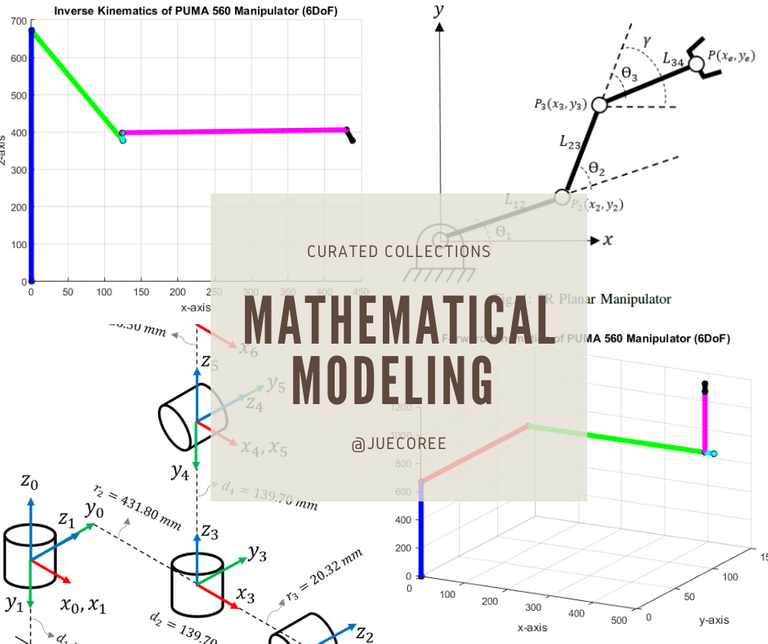# Mathematical Modeling: Kinematics of Robot Manipulators

in Curated Collections2 months ago (edited)### These are collection of articles that explains the mathematical modeling of robot manipulators.

https://peakd.com/hive-196387/@juecoree/forward-and-reverse-kinematics-for-3r-planar-manipulator

The blog gives us a basic understanding on the mathematical modeling of the forward and reverse kinematics of a 3R planar manipulator with the MATLAB implementation.

The 3R planar manipulator has three revolute joint and three links. The robot forward kinematics yields the end-effector position and its orientation from the given link lengths and joint angles. On the contrary, the robot inverse kinematics finds the joint angles from the given end-effector and its orientation.
The blog explained how to derived the forward and inverse kinematics models for a 3R planar manipulator. A correct model must generate the accurate end-effector location and orientation and the joint angles. The blog provided examples that were cross-validated to verify the correctness of each model. The examples in the blog generate the joint angles in the inverse kinematics model, either elbow-up or elbow-down configuration, produce the same end-effector point and orientation.

https://peakd.com/hive-163521/@juecoree/forward-kinematics-for-3r-manipulator-using-dh-method

The blog explained how to obtain the forward kinematics of a robotic arm with three links using Denavit-Hartenberg (DH) representation.

The given parameters in forward kinematics are L1,L2,L3,θ1,θ2 and θ3 then we are required to solve for the location and coordinates of the end-effector (x,y) using Denavit-Hartenberg (DH) convention. The end-effector (x,y) is any device at the end of robotic arm. The Denavit-Hartenberg follows these three basic rules for determining and establishing each coordinate frame. The rules are: The Z_(i-1)axis lies along the axis of motion of the ith joint. (2) The X_i axis is normal to the Z_(i-1)axis and pointing away from it. (3) The Y_i axis completes the right-handed coordinate system as required.
The Denavit-Hartenberg convention represents both translation and rotation. It allows us to have an easier way to find the mathematical models of robot manipulators for a sophisticated robotics system that incorporates translation and rotation.

https://peakd.com/hive-196387/@juecoree/forward-kinematics-of-puma-560-robot-using-dh-method

The blog discussed the steps involved in creating the mathematical model of the forward kinematics of PUMA 560 robot manipulator using DH Method.

Programmable Universal Machine for Assemble (PUMA) 560 robot manipulator is a industrial robotic arm with 6 revolute joint and 6 links. The mathematical model of the forward kinematics of PUMA 560 robot manipulator has 6 degree of freedom and involves the rotation and displacement of its coordinate frames. The forward kinematic analysis of PUMA 560 robot manipulator becomes easier when we use Denavit–Hartenberg (DH) model and general homogeneous transformation matrix.
The DH model allows us to have the forward kinematics of the PUMA 560 robot manipulator. We need to correctly define the DH parameters for the PUMA 560 robot manipulator to get the correct mathematical model.

https://peakd.com/hive-196387/@juecoree/inverse-kinematics-of-puma-560-robot

The blog discussed the derivation of the inverse kinematic of a PUMA 560 robot manipulator by decoupling method.

The mathematical model of the inverse kinematics of PUMA 560 robot manipulator defines the joint angles at each joint for a given transformation matrix T06. The general homogeneous matrix Tn−1n consist the rotation and displacement vectors for a given joint angles.
The Inverse kinematic of 6 degrees of freedom robot manipulator has multiple possible solutions for a given orientation and position of the end-effector. We derived the equations of the joint angles by decoupling the PUMA 560 frames frame 0 to frame 3, and then the last remaining frames.
Sort:

It would be really great if we could push for #Hive front-ends to properly parse LaTeX style equations! A few times now I've started writing math-oriented posts and then just been put off by how awfully ugly it looks and given up.

Posted via proofofbrain.io

Yes, that would be great to have a LaTEX function in hive so that we will have an ease presenting the equations. I usually do the equation in LaTEX and took a screen shot to make it more sleek and clean in the blog. !PIZZA

Congratulations @juecoree! You have completed the following achievement on the Hive blockchain and have been rewarded with new badge(s) :You got more than 2000 replies.Your next target is to reach 2250 replies.

If you no longer want to receive notifications, reply to this comment with the word `STOP`